### Displayed Output

If you use the NOPRINT option in the PROC LOGISTIC statement, the procedure does not display any output. Otherwise, the tables displayed by the LOGISTIC procedure are discussed in the following section in the order in which they appear in the output. Some of the tables appear only in conjunction with certain options or statements; see the section ODS Table Names for details.

Note: The EFFECT, ESTIMATE, LSMEANS, LSMESTIMATE, and SLICE statements also create tables, which are not listed in this section. For information about these tables, see the corresponding sections of Chapter 19: Shared Concepts and Topics.

#### Table Summary

##### Model Information and the Number of Observations

See the section Missing Values for information about missing-value handling, and the sections FREQ Statement and WEIGHT Statement for information about valid frequencies and weights.

##### Response Profile

Displays the Ordered Value assigned to each response level. See the section Response Level Ordering for details.

##### Class Level Information

Displays the design values for each CLASS explanatory variable. See the section Other Parameterizations in Chapter 19: Shared Concepts and Topics, for details.

##### Simple Statistics Tables

The following tables are displayed if you specify the SIMPLE option in the PROC LOGISTIC statement:

• Descriptive Statistics for Continuous Explanatory Variables

• Frequency Distribution of Class Variables

• Weight Distribution of Class VariablesDisplays if you also specify a WEIGHT statement.

##### Strata Tables for (Exact) Conditional Logistic Regression

The following tables are displayed if you specify a STRATA statement:

• Strata SummaryShows the pattern of the number of events and the number of nonevents in a stratum. See the section STRATA Statement for more information.

• Strata InformationDisplays if you specify the INFO option in a STRATA statement.

##### Maximum Likelihood Iteration History

Displays if you specify the ITPRINT option in the MODEL statement. See the sections Iterative Algorithms for Model Fitting, Convergence Criteria, and Existence of Maximum Likelihood Estimates for details.

##### Deviance and Pearson Goodness-of-Fit Statistics

Displays if you specify the SCALE= option in the MODEL statement. Small p-values reject the null hypothesis that the fitted model is adequate. See the section Overdispersion for details.

##### Score Test for the Equal Slopes (Proportional Odds) Assumption

Tests the parallel lines assumption if you fit an ordinal response model with the LINK=CLOGLOG or LINK=PROBIT options. If you specify LINK=LOGIT, this is called the Proportional Odds assumption. The table is not displayed if you specify the UNEQUALSLOPES option in the MODEL statement. Small p-values reject the null hypothesis that the slope parameters for each explanatory variable are constant across all the response functions. See the section Testing the Parallel Lines Assumption for details.

##### Model Fit Statistics

Computes various fit criteria based on a model with intercepts only and a model with intercepts and explanatory variables. If you specify the NOINT option in the MODEL statement, these statistics are calculated without considering the intercept parameters. See the section Model Fitting Information for details.

##### Testing Global Null Hypothesis: BETA=0

Tests the joint effect of the explanatory variables included in the model. Small p-values reject the null hypothesis that all slope parameters are equal to zero,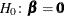. See the sections Model Fitting Information, Residual Chi-Square, and Testing Linear Hypotheses about the Regression Coefficients for details. If you also specify the RSQUARE option in the MODEL statement, two generalized R Square measures are included; see the section Generalized Coefficient of Determination for details.

##### Score Test for Global Null Hypothesis

Displays instead of the Testing Global Null Hypothesis: BETA=0 table if the NOFIT option is specified in the MODEL statement. The global score test evaluates the joint significance of the effects in the MODEL statement. Small p-values reject the null hypothesis that all slope parameters are equal to zero,. See the section Residual Chi-Square for details.

##### Model Selection Tables

The tables in this section are produced when the SELECTION= option is specified in the MODEL statement. See the section Effect-Selection Methods for more information.

• Residual Chi-Square TestDisplays if you specify SELECTION=FORWARD, BACKWARD, or STEPWISE in the MODEL statement. Small p-values reject the null hypothesis that the reduced model is adequate. See the section Residual Chi-Square for details.

• Analysis of Effects Eligible for EntryDisplays if you specify the DETAILS option and the SELECTION=FORWARD or STEPWISE option in the MODEL statement. Small p-values reject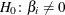. The score chi-square is used to determine entry; see the section Testing Individual Effects Not in the Model for details.

• Analysis of Effects Eligible for RemovalDisplays if you specify the SELECTION=BACKWARD or STEPWISE option in the MODEL statement. Small p-values reject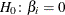. The Wald chi-square is used to determine removal; see the section Testing Linear Hypotheses about the Regression Coefficients for details.

• Analysis of Effects Removed by Fast Backward EliminationDisplays if you specify the FAST option and the SELECTION=BACKWARD or STEPWISE option in the MODEL statement. This table gives the approximate chi-square statistic for the variable removed, the corresponding p-value with respect to a chi-square distribution with one degree of freedom, the residual chi-square statistic for testing the joint significance of the variable and the preceding ones, the degrees of freedom, and the p-value of the residual chi-square with respect to a chi-square distribution with the corresponding degrees of freedom.

• Summary of Forward, Backward, and Stepwise SelectionDisplays if you specify SELECTION=FORWARD, BACKWARD, or STEPWISE in the MODEL statement. The score chi-square is used to determine entry; see the section Testing Individual Effects Not in the Model for details. The Wald chi-square is used to determine removal; see the section Testing Linear Hypotheses about the Regression Coefficients for details.

• Regression Models Selected by Score CriterionDisplays the score chi-square for all models if you specify the SELECTION=SCORE option in the MODEL statement. Small p-values reject the null hypothesis that the fitted model is adequate. See the section Effect-Selection Methods for details.

##### Type 3 Analysis of Effect

Displays if the model contains a CLASS variable. Performs Wald chi-square tests of the joint effect of the parameters for each CLASS variable in the model. Small p-values reject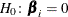. See the section Testing Linear Hypotheses about the Regression Coefficients for details.

##### Analysis of Maximum Likelihood Estimates

CLASS effects are identified by their (nonreference) level. For generalized logit models, a response variable column displays the nonreference level of the logit. The table includes the following:

• the estimated standard error of the parameter estimate, computed as the square root of the corresponding diagonal element of the estimated covariance matrix

• the Wald chi-square statistic, computed by squaring the ratio of the parameter estimate divided by its standard error estimate. See the section Testing Linear Hypotheses about the Regression Coefficients for details.

• the p-value tests the null hypothesis; small values reject the null.

• the standardized estimate for the slope parameter, if you specify the STB option in the MODEL statement. See the STB option for details.

• exponentiated values of the estimates of the slope parameters, if you specify the EXPB option in the MODEL statement. See the EXPB option for details.

• the label of the variable, if you specify the PARMLABEL option in the MODEL statement and if space permits. Due to constraints on the line size, the variable label might be suppressed in order to display the table in one panel. Use the SAS system option LINESIZE= to specify a larger line size to accommodate variable labels. A shorter line size can break the table into two panels allowing labels to be displayed.

##### Odds Ratio Estimates

Displays the odds ratio estimates and the corresponding 95% Wald confidence intervals for variables that are not involved in nestings or interactions. For continuous explanatory variables, these odds ratios correspond to a unit increase in the risk factors. See the section Odds Ratio Estimation for details.

##### Association of Predicted Probabilities and Observed Responses

See the section Rank Correlation of Observed Responses and Predicted Probabilities for details.

##### Parameter Estimates and Profile-Likelihood or Wald Confidence Intervals

Displays if you specify the CLPARM= option in the MODEL statement. See the section Confidence Intervals for Parameters for details.

##### Odds Ratio Estimates and Profile-Likelihood or Wald Confidence Intervals

Displays if you specify the ODDSRATIO statement for any effects with any class parameterizations. Also displays if you specify the CLODDS= option in the MODEL statement, except odds ratios are computed only for main effects not involved in interactions or nestings, and if the main effect is a CLASS variable, the parameterization must be EFFECT, REFERENCE, or GLM. See the section Odds Ratio Estimation for details.

##### Estimated Covariance or Correlation Matrix

Displays if you specify the COVB or CORRB option in the MODEL statement. See the section Iterative Algorithms for Model Fitting for details.

##### Contrast Test Results

Displays the Wald test for each specified CONTRAST statement. Small p-values reject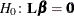. The Coefficients of Contrast table displays the contrast matrix if you specify the E option, and the Contrast Estimation and Testing Results by Row table displays estimates and Wald tests for each row of the contrast matrix if you specify the ESTIMATE= option. See the sections CONTRAST Statement, Testing Linear Hypotheses about the Regression Coefficients, and Linear Predictor, Predicted Probability, and Confidence Limits for details.

##### Linear Hypotheses Testing Results

Displays the Wald test for each specified TEST statement. See the sections Testing Linear Hypotheses about the Regression Coefficients and TEST Statement for details.

##### Hosmer and Lemeshow Goodness-of-Fit Test

Displays if you specify the LACKFIT option in the MODEL statement. Small p-values reject the null hypothesis that the fitted model is adequate. The Partition for the Hosmer and Lemeshow Test table displays the grouping used in the test. See the section The Hosmer-Lemeshow Goodness-of-Fit Test for details.

##### Classification Table

Displays if you use the CTABLE option in the MODEL statement. If you specify a list of cutpoints with the PPROB= option, then the cutpoints are displayed in the Prob Level column. If you specify the prior event probabilities with the PEVENT= option, then the probabilities are displayed in the Prob Event column. The Correct column displays the number of correctly classified events and nonevents, the Incorrect Event column displays the number of nonevents incorrectly classified as events, and the Incorrect Nonevent column gives the number of nonevents incorrectly classified as events. See the section Classification Table for more details.

##### Regression Diagnostics

Displays if you specify the INFLUENCE option in the MODEL statement. See the section Regression Diagnostics for more information about diagnostics from an unconditional analysis, and the section Regression Diagnostic Details for information about diagnostics from a conditional analysis.

##### Fit Statistics for SCORE Data

Displays if you specify the FITSTAT option in the SCORE statement. See the section Scoring Data Sets for details.

##### ROC Association Statistic and Contrast Tables

Displayed if a ROC statement or a ROCCONTRAST statement is specified. See the section ROC Computations for details about the Mann-Whitney statistics and the test and estimation computations, and see the section Rank Correlation of Observed Responses and Predicted Probabilities for details about the other statistics.

##### Exact Conditional Logistic Regression Tables

The tables in this section are produced when the EXACT statement is specified. If the METHOD=NETWORKMC option is specified, the test and estimate tables are renamed Monte Carlo tables and a Monte Carlo standard error column (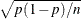) is displayed.

• Sufficient StatisticsDisplays if you request an OUTDIST= data set in an EXACT statement. The table lists the parameters and their observed sufficient statistics.

• (Monte Carlo) Conditional Exact TestsSee the section Hypothesis Tests for details.

• (Monte Carlo) Exact Parameter EstimatesDisplays if you specify the ESTIMATE option in the EXACT statement. This table gives individual parameter estimates for each variable (conditional on the values of all the other parameters in the model), confidence limits, and a two-sided p-value (twice the one-sided p-value) for testing that the parameter is zero. See the section Inference for a Single Parameter for details.

• (Monte Carlo) Exact Odds RatiosDisplays if you specify the ESTIMATE=ODDS or ESTIMATE=BOTH option in the EXACT statement. See the section Inference for a Single Parameter for details.